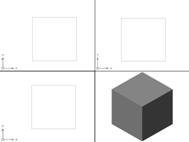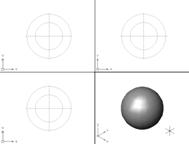• 用Matplotlib画三维图最基本的三维图是由(x, y, z)三维坐标点构成的线图与散点图，可以用ax.plot3D和ax.scatter3D函数来创建，默认情况下，散点会自动改变透明度，以在平面上呈现出立体感三维的线图和散点图#绘制...
用Matplotlib画三维图最基本的三维图是由(x, y, z)三维坐标点构成的线图与散点图，可以用ax.plot3D和ax.scatter3D函数来创建，默认情况下，散点会自动改变透明度，以在平面上呈现出立体感三维的线图和散点图#绘制三角螺旋线from mpl_toolkits import mplot3d%matplotlib inlineimport matplotlib.pyplot as pltimport numpy as npax = plt.axes(projection='3d')#三维线的数据zline = np.linspace(0, 15, 1000)xline = np.sin(zline)yline = np.cos(zline)ax.plot3D(xline, yline, zline, 'gray')# 三维散点的数据zdata = 15 * np.random.random(100)xdata = np.sin(zdata) + 0.1 * np.random.randn(100)ydata = np.cos(zdata) + 0.1 * np.random.randn(100)ax.scatter3D(xdata, ydata, zdata, c=zdata, cmap='Greens')三维等高线图def f(x, y):return np.sin(np.sqrt(x ** 2 + y ** 2))x = np.linspace(-6,6,30)y = np.linspace(-6,6,30)X, Y = np.meshgrid(x, y)Z = f(X,Y)fig = plt.figure()ax = plt.axes(projection='3d')ax.contour3D(X, Y, Z, 50, cmap='binary')ax.set_xlabel('x')ax.set_ylabel('y')ax.set_zlabel('z')#调整观察角度和方位角。这里将俯仰角设为60度，把方位角调整为35度ax.view_init(60, 35)线框图和全面图全面图和线框图相似，只不过线框图的每一个面都是由多边形构成。只要增加唉一个配色方案来填充这些多边形，就可以感受到可视化图形表面的拓扑结构了。#线框图fig =plt.figure()ax = plt.axes(projection='3d')ax.plot_wireframe(X, Y, Z, color='c')ax.set_title('wireframe')#曲面图ax = plt.axes(projection='3d')ax.plot_surface(X, Y, Z, rstride=1, cstride=1, cmap='viridis', edgecolor='none')ax.set_title('surface')#使用极坐标可以获得切片的效果r = np.linspace(0, 6, 20)theta = np.linspace(-0.9 * np.pi, 0.8 * np.pi, 40)r, theta = np.meshgrid(r, theta)X = r * np.sin(theta)Y = r * np.cos(theta)Z = f(X, Y)ax = plt.axes(projection='3d')ax.plot_surface(X, Y, Z, rstride=1, cstride=1, cmap='viridis', edgecolor='none')曲面三角剖分在某些应用场景下，上述这些要求均匀采样的网格数据显得太过严格且不太容易实现。这时就可以使用三角剖分部分图形。theta = 2 * np.pi * np.random.random(1000)r = 6 * np.random.random(1000)x = np.ravel(r * np.sin(theta))y = np.ravel(r * np.cos(theta))z = f(x, y)ax = plt.axes(projection='3d')ax.scatter(x, y, z, c=z, cmap='viridis', linewidth=0.5)#上图还有许多地方需要修补，这些工作可以由ax.plot_trisurf函数帮助我们完成。它首先找到一组所有点都连接起来的三角形，然后用这些三角形创建曲面ax = plt.axes(projection='3d')ax.plot_trisurf(x, y, z, cmap='viridis', edgecolor='none')莫比乌斯带（应用曲面三角剖分）#绘制莫比乌斯带#由于它是一条二维带，因此需要两个内在维度。theta维度取值范围是0～2pi，宽度维度w取值范围是-1～1theta = np.linspace(0, 2 * np.pi, 30)w = np.linspace(-0.25, 0.25, 8)w, theta = np.meshgrid(w, theta)phi = 0.5 * theta#x-y平面内的半径r = 1 + w * np.cos(phi)x = np.ravel(r * np.cos(theta))y = np.ravel(r * np.sin(theta))z = np.ravel(w * np.sin(phi))#要画出莫比乌斯带，还必须保证三角部分是正确的。最好的方法是首先用基本参数化方法定义三角部分，然后用Matplotlib将#这个三角剖分映射到莫比乌斯带的三维空间里from matplotlib.tri import Triangulationtri = Triangulation(np.ravel(w), np.ravel(theta))ax = plt.axes(projection='3d')ax.plot_trisurf(x, y, z, triangles=tri.triangles, cmap='viridis', linewidth=0.2)ax.set_xlim(-1, 1);ax.set_ylim(-1,1);ax.set_zlim(-1,1)到此这篇关于如何用Matplotlib 画三维图的示例代码的文章就介绍到这了,更多相关Matplotlib 三维图内容请搜索脚本之家以前的文章或继续浏览下面的相关文章希望大家以后多多支持脚本之家！
展开全文• 用Matplotlib画三维图最基本的三维图是由(x, y, z)三维坐标点构成的线图与散点图，可以用ax.plot3D和ax.scatter3D函数来创建，默认情况下，散点会自动改变透明度，以在平面上呈现出立体感三维的线图和散点图#绘制...
用Matplotlib画三维图最基本的三维图是由(x, y, z)三维坐标点构成的线图与散点图，可以用ax.plot3D和ax.scatter3D函数来创建，默认情况下，散点会自动改变透明度，以在平面上呈现出立体感三维的线图和散点图#绘制三角螺旋线from mpl_toolkits import mplot3d%matplotlib inlineimport matplotlib.pyplot as pltimport numpy as npax = plt.axes(projection='3d')#三维线的数据zline = np.linspace(0, 15, 1000)xline = np.sin(zline)yline = np.cos(zline)ax.plot3D(xline, yline, zline, 'gray')# 三维散点的数据zdata = 15 * np.random.random(100)xdata = np.sin(zdata) + 0.1 * np.random.randn(100)ydata = np.cos(zdata) + 0.1 * np.random.randn(100)ax.scatter3D(xdata, ydata, zdata, c=zdata, cmap='Greens')三维等高线图def f(x, y):return np.sin(np.sqrt(x ** 2 + y ** 2))x = np.linspace(-6,6,30)y = np.linspace(-6,6,30)X, Y = np.meshgrid(x, y)Z = f(X,Y)fig = plt.figure()ax = plt.axes(projection='3d')ax.contour3D(X, Y, Z, 50, cmap='binary')ax.set_xlabel('x')ax.set_ylabel('y')ax.set_zlabel('z')#调整观察角度和方位角。这里将俯仰角设为60度，把方位角调整为35度ax.view_init(60, 35)线框图和全面图全面图和线框图相似，只不过线框图的每一个面都是由多边形构成。只要增加唉一个配色方案来填充这些多边形，就可以感受到可视化图形表面的拓扑结构了。#线框图fig =plt.figure()ax = plt.axes(projection='3d')ax.plot_wireframe(X, Y, Z, color='c')ax.set_title('wireframe')#曲面图ax = plt.axes(projection='3d')ax.plot_surface(X, Y, Z, rstride=1, cstride=1, cmap='viridis', edgecolor='none')ax.set_title('surface')#使用极坐标可以获得切片的效果r = np.linspace(0, 6, 20)theta = np.linspace(-0.9 * np.pi, 0.8 * np.pi, 40)r, theta = np.meshgrid(r, theta)X = r * np.sin(theta)Y = r * np.cos(theta)Z = f(X, Y)ax = plt.axes(projection='3d')ax.plot_surface(X, Y, Z, rstride=1, cstride=1, cmap='viridis', edgecolor='none')曲面三角剖分在某些应用场景下，上述这些要求均匀采样的网格数据显得太过严格且不太容易实现。这时就可以使用三角剖分部分图形。theta = 2 * np.pi * np.random.random(1000)r = 6 * np.random.random(1000)x = np.ravel(r * np.sin(theta))y = np.ravel(r * np.cos(theta))z = f(x, y)ax = plt.axes(projection='3d')ax.scatter(x, y, z, c=z, cmap='viridis', linewidth=0.5)#上图还有许多地方需要修补，这些工作可以由ax.plot_trisurf函数帮助我们完成。它首先找到一组所有点都连接起来的三角形，然后用这些三角形创建曲面ax = plt.axes(projection='3d')ax.plot_trisurf(x, y, z, cmap='viridis', edgecolor='none')莫比乌斯带（应用曲面三角剖分）#绘制莫比乌斯带#由于它是一条二维带，因此需要两个内在维度。theta维度取值范围是0～2pi，宽度维度w取值范围是-1～1theta = np.linspace(0, 2 * np.pi, 30)w = np.linspace(-0.25, 0.25, 8)w, theta = np.meshgrid(w, theta)phi = 0.5 * theta#x-y平面内的半径r = 1 + w * np.cos(phi)x = np.ravel(r * np.cos(theta))y = np.ravel(r * np.sin(theta))z = np.ravel(w * np.sin(phi))#要画出莫比乌斯带，还必须保证三角部分是正确的。最好的方法是首先用基本参数化方法定义三角部分，然后用Matplotlib将#这个三角剖分映射到莫比乌斯带的三维空间里from matplotlib.tri import Triangulationtri = Triangulation(np.ravel(w), np.ravel(theta))ax = plt.axes(projection='3d')ax.plot_trisurf(x, y, z, triangles=tri.triangles, cmap='viridis', linewidth=0.2)ax.set_xlim(-1, 1);ax.set_ylim(-1,1);ax.set_zlim(-1,1)到此这篇关于如何用Matplotlib 画三维图的示例代码的文章就介绍到这了,更多相关Matplotlib 三维图内容请搜索脚本之家以前的文章或继续浏览下面的相关文章希望大家以后多多支持脚本之家！
展开全文• 各位兄弟姐妹，最近准备在程序中一个比较简单的三维曲面图，就是根据一个矩阵三维图形出来，网格图也可以。要怎么，各位能否给个建议？？非常感谢！！
当我们在使用CAD软件绘制图纸时，为了更加直观的了解实体对象的构成及立体构造，我们就会需要绘制三维实体对象。那在浩辰CAD软件中画三维图的时候，如何绘制三维实体对象呢？今天就为大家简单介绍下。CAD中绘制三维实体的过程：3.1长方体1.命令格式命令行：Box菜单：[绘图]→[实体]→[长方体(B)]工具栏：[实体]→[长方体]创建三维长方体对象。2.操作步骤创建边长都为10的立方体，如图13-13。图13-13用Box命令绘制立方体命令:Box执行Box命令指定长方体的角点或[中心(C)] <0,0,0>:点取一点指定图形的一个角点指定角点或[立方体(C)/长度(L)]: @10,10指定XY平面上矩形大小长方体高度:10指定高度，回车结束命令以上各选项含义和功能说明如下：长方体的角点：指定长方体的第一个角点。中心(C)：通过指定长方体的中心点绘制长方体。立方体(C)：指定长方体的长、宽、高都为相同长度。长度(L)：通过指定长方体的长、宽、高来创建三维长方体。3.注意@若输入的长度值或坐标值是正值，则以当前UCS坐标的X、Y、Z轴的正向创建立图形；若为负值，则以X、Y、Z轴的负向创建立图形。13.3.2球体1.命令格式命令行：Sphere菜单：[绘图]→[实体]→[球体(S)]工具栏：[实体]→[球体]绘制三维球体对象。默认情况下，球体的中心轴平行于当前用户坐标系(UCS)的Z轴。纬线与XY平面平行。2.操作步骤创建半径为10的球体，如图13-14。图13-14用Sphere命令创建球体命令:Sphere执行Sphere命令球体中心:点选一点指定球心位置指定球体半径或[直径(D)]:10指定半径值，回车结束命令以上各选项含义和功能说明如下：球体半径(R)：绘制基于球体中心和球体半径的球体对象。直径(D)：绘制基于球体中心和球体直径的球体对象。以上就是在浩辰CAD软件中，当我们需要通过绘制三维实体对象来了解实体的空间效果时，我们就需要在画三维图的时候，绘制三维实体。今天就介绍这么多了。安装浩辰CAD软件试试吧。更多CAD教程技巧，可关注浩辰CAD官网进行查看。
展开全文• 你好。运行一下这个代码试试3D绘图示意import mpl_toolkits.mplot3d import Axes3Dfig = plt.figure()ax = Axes3D(fig)x = np.arange(-4, 4, 0.25)y = np.arange(-4, 4, 0.25)x, y = np.meshgrid(x, y)r = np.sqrt...
你好。运行一下这个代码试试3D绘图示意import mpl_toolkits.mplot3d import Axes3Dfig = plt.figure()ax = Axes3D(fig)x = np.arange(-4, 4, 0.25)y = np.arange(-4, 4, 0.25)x, y = np.meshgrid(x, y)r = np.sqrt(x2 + y2)z = np.sin(r)ax.plot_surface(x, y, z, rstride = 1, # row 行步长cstride = 2, # colum 列步长cmap=plt.cm.hot ) # 渐变颜色ax.contourf(x, y, z,zdir='z', # 使用数据方向offset=-2, # 填充投影轮廓位置cmap=plt.cm.hot)ax.set_zlim(-2, 2)plt.show()
展开全文• 他可以绘制各种图形，可是最近最的一个小程序，得到一些三维的数据点图，就学习了下python中的matplotlib模块，如何绘制三维图形。初学者，可能对这些第三方库安装有一定的小问题，对于一些安装第三方库经验较少的...
• ）于是，重新整理、注释了一下代码，下载方式见链接中文章的末尾，需要的自取~如何用Matlab在论文中出漂亮的插图​mp.weixin.qq.com----以下原回答----翻遍这个问题下的所有回答，发现凡是提到Matlab的，其评价中...
• 三维动画从表现形式上可分的...三维动画制作-水木动画 首先是三维动画造型具有角色形象性和空间立体感两大特征。角色造型主要分为拟人化造型与写意造型两大类；角色造型设计要重视风格设计、角色组合、资源挖掘、...
• 今天就来分享下如何一步步绘制出三维矢量（SVG）图。八面体我们先以下面这个八面体为例。1 安装相关包首先安装两个必备包：import pyrr # NumPy 的 3D 函数库import svgwrite # svg图形处理库2 定义 3D 图生成环境接...
• 今天就来分享下如何一步步绘制出三维矢量（SVG）图。八面体我们先以下面这个八面体为例。 1 安装相关包首先安装两个必备包：importpyrr# NumPy 的 3D 函数库importsvgwrite# svg图形处理库2 定义 3D...
• 三维立体给人一种空间感，在视觉上更美观灵动，通常我们说的三维是指在平面二维系中又加入了一个方向向量构成...那么实现Vegas三维立体如何调整呢？更多效果可以点击：Vegas 对静态图片做动画效果。http://www.veg...
• 之前教了大家不少简单的画图方法...如果需要绘制三维的图形，matlab可以，python同样可以，代码风格也是极其的与matlab类似。importnumpyasnpimportmatplotlib.pyplotaspltfrommatplotlibimportcmfrommpl_toolkits.m...
• 用Matplotlib画三维图最基本的三维图是由(x, y, z)三维坐标点构成的线图与散点图，可以用ax.plot3D和ax.scatter3D函数来创建，默认情况下，散点会自动改变透明度，以在平面上呈现出立体感三维的线图和散点图#绘制...
• 今天就来分享下如何一步步绘制出三维矢量（SVG）图。八面体我们先以下面这个八面体为例。1 安装相关包首先安装两个必备包：import pyrr # NumPy 的 3D 函数库import svgwrite # svg图形处理库2 ...
• 最基本的三维图是由(x, y, z)三维坐标点构成的线图与散点图，可以用ax.plot3D和ax.scatter3D函数来创建，默认情况下，散点会自动改变透明度，以在平面上呈现出立体三维的线图和散点图...toolkitsimport mplot3d%...
• 用Matplotlib画三维图 最基本的三维图是由(x, y, z)三维坐标点构成的线图与散点图，可以用ax.plot3D和ax.scatter3D函数来创建，默认情况下，散点会自动改变透明度，以在平面上呈现出立体感 三维的线图和散点图 #...
• 用Matplotlib画三维图最基本的三维图是由(x, y, z)三维坐标点构成的线图与散点图，可以用ax.plot3D和ax.scatter3D函数来创建，默认情况下，散点会自动改变透明度，以在平面上呈现出立体感三维的线图和散点图#绘制...matlab
• 用Matplotlib画三维图最基本的三维图是由(x, y, z)三维坐标点构成的线图与散点图，可以用ax.plot3D和ax.scatter3D函数来创建，默认情况下，散点会自动改变透明度，以在平面上呈现出立体感三维的线图和散点图#绘制...
• 首先介绍一个函数ellipsoid,这个函数是在三维立体空间椭圆用的这个函数参数如下：ellipsoid(x,y,z,x1,y1,z1) x,y,z代表了椭球的中心,x1,y1,z1代表了x,y,z方向的分量然后如果是要球的话，把这三个分量全部都写成...函数 matlab 数据 as
• 原标题：Python 竟能绘制出如此酷炫的三维图作者 ...今天就来分享下如何一步步绘制出三维矢量（SVG）图。八面体我们先以下面这个八面体为例。 1 安装相关包首先安装两个必备包：importpyrr # NumPy 的 3D 函数库impo...
• Surfer 软件是美国Golden Software 公司编制的一款画三维图（等高线，image map, 3d surface）的软件，这款软件是非常不错的三维立体图制作软件，也是地质工作者必备的专业成图软件。该软件简单易学，可以在几分钟内...surfer
• 众所周知，3D设计是一门专业性很强的技术，对于特定的设计有着高标准的要求，学会这...3D模型呈现出的三维立体效果给人一种真实可靠的形象体验，我们时常会感叹拥有此项技术的人员是多么神器，竟然能创造这种感觉。学会